# AP Calculus BC Practice Test 31

### Test Information10 questions20 minutes

Calculator Disallowed

1.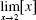(where [x] is the greatest integer in x) is

2.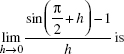3.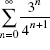4. The equation of the tangent to the curve 2x2 - y4 = 1 at the point (-1, 1) is

5. The nth term of the Taylor series expansion about x = 0 of the function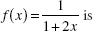6. When the method of partial fractions is used to decompose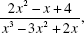one of the fractions obtained is

7. A relative maximum value of the function is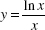is

8. When a series is used to approximate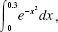the value of the integral, to two decimal places, is

9.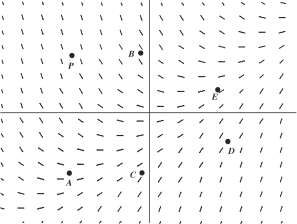A particular solution of the differential equation whose slope field is shown abovecontains point P. This solution may also contain which other point?

10. Let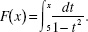Which of the following statements is (are) true?

I. The domain of F is x ≠ ±1.

II. F(2) > 0.

III. The graph of F is concave upward.